## A Calibration Manager's Guide to Measurement Uncertainties

 Live chat hosted software Our online text chat service enables you to contact us in real-time. We will be pleased to discuss all aspects of our software with you. Please click on the link on the left to contact us. If no operator is available, you will be able to e-mail us.

### Introduction

Performing calibrations is an accurate and demanding task. We work with the best instruments at our disposal, ensuring that everything we use is traceably calibrated. We ensure that all our measurements are taken in accordance with appropriate procedures and methods, and that all data is clearly and accurately recorded and presented.

We do not, however, live in a perfect world. We must be honest both to ourselves and to those using the calibration data that we produce. A key element of this honesty is an accurate statement of measurement uncertainty. Some calibration engineers mistakenly view uncertainties as a measure of the accuracy of their work, and the diligence with which it was performed. Nothing could be further from the truth. It its merely an acceptance of the fact that perfection can be strived for but never attained.

Measurement Uncertainties are nothing new. When I was a young Physics undergraduate, over 25 years ago, no reported measurement would be considered complete unless it was accompanied by a statement of "experimental errors" as they were called in those days. They are now referred to as "measurement uncertainties" for 2 reasons:

1. The word "error" now has another specific use: the numerical difference between the actual value (the measurand) and the observed value of the measurand. This does not include:

• Misreading of instruments such as analogue gauges
• Failure to apply known correction factors to raw data
• Incorrect recording or misinterpretation of experimental data
These are simply mistakes that must be avoided.

2. As we mentioned earlier, people do not feel comfortable with the word "error" since it can be misinterpreted as an assesment of the quallity of their work.

 Terminology Definition The Measurand This is the actual value of the quantity we are attempting measure. The Observed Value The value that we measure as our interpretation of the measurand. Measurement Error This is the difference between the Observed Value and the Measurand.

An overall measurement uncertainty will consist of contributions from a number of components. Some of these components are estimated on the basis of the statistical distribution of a series of measurements and are characterized by experimental standard deviations. These are called Type A evaluation, and are discussed in detail in other article on this website.

Another type of uncertainty component is known as Type B. Values for Type B evaluation are based on information obtained from either an outside source, or scientific judgment and experience based on all available information. Values belonging in this category may be derived from:

• Previous measurement data.
• Experience with or general knowledge of the behavior and properties of relevant materials and instruments.
• Manufacturer’s specifications.
• Data provided in calibration and other certificates.
• Uncertainties assigned to reference data taken from handbooks.

The measurement uncertainty for any given calibration is the combination of all the Type A and Type B uncertainty components that occur in the calibration.

The first stage to obtaining an overall (or "combined") uncertainty of a calibration is to list all of the instruments that are used in the calibration. Next you must measure, or, more likely obtain from the manufacturer, all uncertainty values for each instrument, together with either a "coverage factor" or probalbility distribution, both of which are explained later.

You must also include in your list any other factors (ambient temperature, relative humidity, electromagnetic interference, etc) that might influence result of your calibration. The completed list is called an "Uncertainty Budget".

### Type B Uncertainties

In practice you will find that there are only 2 kinds of Type B uncertainty in common use:

• Uncertainty value obtained from an outside source
• Uncertainty value obtained from an assumed probability distribution

Since contributions obtained from an outside source are pretty straight-forward, let us now look at values obtained from an assumed probability distribution in more detail:

Have a look at the picture below. The graphs represent the results of a large number of repeat measurements of a value. The horizontal axis represents the measured value and the vertical axis represents the number of measurements with that value.

In all 3 diagrams we are making a series of repeat measurements of a parameter whose actual value is M (the measurand). We know that the tolerance on our measurement is ±a. So all measurements (correctly made) will lie between the vales of M+a and M-a. Now, if it is just as likely for a measurement to be any value between these limits, we would say that its probabiity distribtion is "rectangular" (sometimes also known as "uniform") as in the centre picture.

A triangular or normal distribution would be used when the values of the quantity are known to be more likely near the center of the limits. These are shown in the left and right-hand pictures.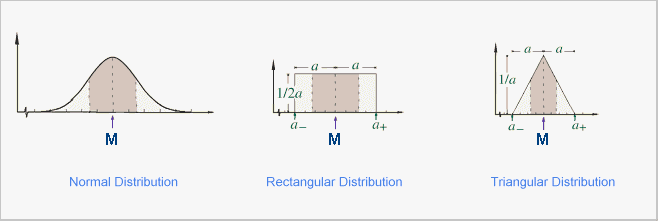In all 3 cases, we should expect that the mean of all our measurements will be the value M, that of our measurand. If it is not, there is some systematic error that needs to be eliminated or compensated for.

### Estimating Type B Uncertainties

The first thing we need to do is to work out the limits of our measurement. For example a length measurement made with a steel rule will be something like ±0.5mm.

Next, you will need to find out which probability distribution best fits your measurement, in the case above, rectangular is as good as any. Based on this decision you will then multiply your limit value by a factor which produces your Type B uncertainty value. (The derivation of each factor is based on statistical analysis and will not be covered here). The various options are shown below:

 Calculating Standard Uncertainty Uj Data given An uncertainty given by a manufacturer or provided on a certificate with a Coverage Factor = 1 Uj is the given value Data given An uncertainty given by a manufacturer or provided on a certificate with a Coverage Factor = 2 Divide the given value by 2 to obtain Uj Normal distribution: "1 out of 2" Model the input quantity in question by a normal probability distribution and estimate lower and upper limits a- and a+ such that the best estimated value of the input quantity is (a+ + a-)/2 (i.e., the center of the limits) and there is 1 chance out of 2 (i.e., a 50 % probability) that the value of the quantity lies in the interval a- to a+. Then uj is approximately 1.48 a, where a = (a+ - a-)/2 is the half-width of the interval. Normal distribution: "2 out of 3" Model the input quantity in question by a normal probability distribution and estimate lower and upper limits a- and a+ such that the best estimated value of the input quantity is (a+ + a-)/2 (i.e., the center of the limits) and there are 2 chances out of 3 (i.e., a 67 % probability) that the value of the quantity lies in the interval a- to a+. Then uj is approximately a, where a = (a+ - a-)/2 is the half-width of the interval. Normal distribution: "99.73 %" If the quantity in question is modeled by a normal probability distribution, there are no finite limits that will contain 100 % of its possible values. However, plus and minus 3 standard deviations about the mean of a normal distribution corresponds to 99.73 % limits. Thus, if the limits a- and a+ of a normally distributed quantity with mean (a+ + a-)/2 are considered to contain "almost all" of the possible values of the quantity, that is, approximately 99.73 % of them, then uj is approximately a/3, where a = (a+ - a-)/2 is the half-width of the interval. Uniform (rectangular) distribution Estimate lower and upper limits a- and a+ for the value of the input quantity in question such that the probability that the value lies in the interval a- and a+ is, for all practical purposes, 100 %. Provided that there is no contradictory information, treat the quantity as if it is equally probable for its value to lie anywhere within the interval a- to a+; that is, model it by a uniform (i.e., rectangular) probability distribution. The best estimate of the value of the quantity is then (a+ + a-)/2 with uj = a divided by the square root of 3, where a = (a+ - a-)/2 is the half-width of the interval. Triangular distribution Estimate lower and upper limits a- and a+ for the value of the input quantity in question such that the probability that the value lies in the interval a- to a+ is, for all practical purposes, 100 %. Provided that there is no contradictory information, model the quantity by a triangular probability distribution. The best estimate of the value of the quantity is then (a+ + a-)/2 with uj = a divided by the square root of 6, where a = (a+ - a-)/2 is the half-width of the interval.

### Combining Uncertainties

Having obtained your individual standard uncertainties, you will now need to combine them all together to produce a combined uncertainty estimate for your calibration.

To do this, we add all of the individual components together "in quadrature" in other words, add together the squares of all the individual standard uncertainties, and take the square root of the total. This method assumes one basic fact: that there is no connection between the individual components i.e. that a change in one has no effect on any other. This will more often than not be the case. This is best illustrated by an example:

In a simple temperature calibration 2 instruments are used: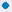A calibration block - standard uncertainty 20 mKelvin, coverage factor = 2 Uj = 20 ÷ 2 = 10A reference thermometer - standard uncertainty 10 mKelvin, coverage factor = 2 Uj = 10 ÷ 2 = 5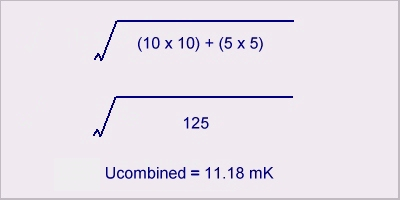The Calibration Toolbox Uncertainty Calculator There is nowadays an increasing requirement to provide a statement of the statistical uncertainty associated with an individual measurement. The Calibration Toolbox Uncertainty Calculator calculator enables you to calculate Type A uncertainties and to combine Type B uncertainty analysis with the means of calculating the combined uncertainty of an number of individual uncertainty contributions by means of a detailed error budget. There are a number of illustrative examples available for you to look at.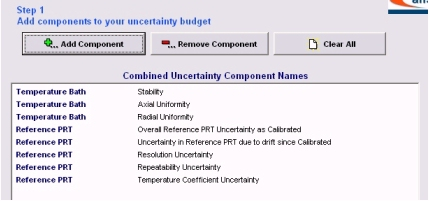Once you have created your list of uncertainty components, you enter the given values for Uj and select the probability distribution for each from a drop down list. The caclulator will then calculate the combined uncertainty for you.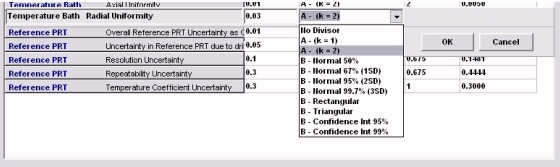We have discussed how the values of uncertainty contributions can be obtained from a variety of sources. It may well be the case that a manufacturer will supply a single uncertainty value for an instrument, whereas others may provide the individual contributions that go to make up that value. In all cases, however, 2 pieces of information will be provided: a numerical value and some indication of the associated statistical distribution. A detailed description of the statistical aspects is beyond the scope of this article. There are several good reference sources on the Internet, try visiting the NIST website at: http://physics.nist.gov/cuu/ The Calibration Toolbox Uncertainty Calculator means that you do not need a detailed knowledge of statistics. It suffices to understand that, the uncertainties quoted need to be multiplied by some sort of factor before they can be included into a combined value. Most uncertainty values represent some sort of standard deviation. The factor to be used will depend on the theoretical probability distribution associated with the value (normal, rectangular or triangular), or it’s coverage factor.

### Coverage Factors

Let's take another look at the probability distribution graphs that we examined earlier:These represent the results of a large number of repeat measurements of a value. The horizontal axis represents the measured value and the vertical axis represents the number of measurements with that value. The shaded areas represent the number of readings that lie within ( ±1 x Standard Uncertainty ), in the case of the normal distribution this is approximately 65% of the measurements.

If we now imagine that we double the width of the shaded band, say to ( ±2 x Standard Uncertainty ), it is clear that more of the sample will fall into the new shaded area. In fact for this case we will have over 95% of our sample within the area. If we now go to ( ±3 x Standard Uncertainty ) we have 99% etc.

The multiples that we use (e.g. 1, 2 and 3) are known as Coverage Factors and are used to express what percentage of the sample will be covered by our band. The associated percentages are referred to as the Confidence Interval

### Expression of Uncertainties

There is no prescribed method for reporting uncertainties. Statements need to be clar and concise. Here are a few good examples:REFERENCE STANDARD:The mass of the weight under test was measured as 10,000.005 g with an associated expanded uncertainty of 45 mg (coverage factor k=2), Type B rectangular distribution.The reported uncertainty is based on a standard uncertainty multiplied by a coverage factor k = 2, which provides a confidence level of approximately 95 %. The standard uncertainty has been determined in accordance with EA 04/2.Uncertainty statement: The combined standard uncertainty includes the standard uncertainty reported for the standard, the standard uncertainty for the measurement process, the standard uncertainty for any uncorrected errors associated with buoyancy corrections, and a component of uncertainty to account for any observed deviations from NIST values that are less than surveillance limits. The combined standard uncertainty is multiplied by a coverage factor of 2 to give an expanded uncertainty, which defines an interval having a level of confidence of approximately 95 percent. The expanded uncertainty presented in this report is consistent with the 1993 ISO Guide to the Expression of Uncertainty in Measurement. The expanded uncertainty is not to be confused with a tolerance limit for the user during application.

### Best Measurement Capability

Best measurement capability (always referring to a particular quantity, the measurand) is defined as the smallest uncertainty of measurement that a laboratory can achieve within its scope of accreditation, when performing more or less routine calibrations of nearly ideal measurement standards or measuring instruments designed for the measurement of that quantity. The assessment of best measurement capability of accredited calibration laboratories is based on the method described in the accreditation document, and will normally be supported or confirmed by experimental evidence.

Again it is a fact of life that reducing measurement uncertainties costs money, but a well performed uncertainty budget will indicate where uncertainties can be reduced at least cost.

 Acknowledgement is given to: NIST Technical Document 1297 Guidelines for Evaluating and Expressing the Uncertainty of NIST Measurement Results.